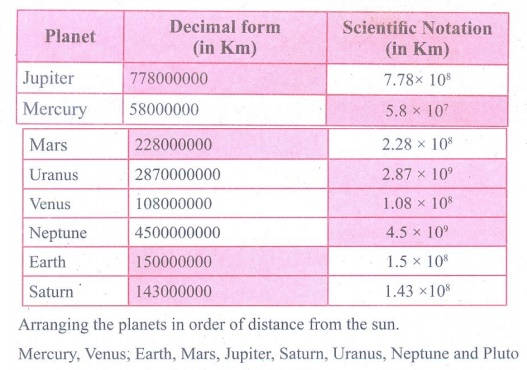Home | | Maths 9th std | Student Activity Questions and Answers

# Student Activity Questions and Answers

Maths : Real Numbers: Book Back, Exercise, Example Numerical Question with Answers, Solution : Student Activity Questions and Answers

Activity – 1

Is it interesting to see this pattern ?Verify it. Can you frame 4 such new surds?

Solution:Activity – 2

Take a graph sheet and mark OABC as follows.In the square OABC,

OA = AB = BC = OC = 1unit

Consider right angled DOAC

AC = √[12 +12]

= √2 unit [By Pythagoras theorem]

The length of the diagonal (hypotenuse)

AC = √2 , which is a surd.

Consider the following graphs:Let us try to find the length of AC in two different ways :

(diagonals of units squares)

2+2+2

AC = 32 units

AC = √[OA2 +OC2] = √[32 +32]

√[9 + 9 ]

AC = 18 units

Are they equal? Discuss. Can you verify the same by taking different squares of different lengths?

Solution:Activity − 3

The following list shows the mean distance of the planets of the solar system from the Sun. Complete the following table. Then arrange in order of magnitude starting with the distance of the planet closest to the Sun.Solution:Tags : Numerical Problems with Answers, Solution | Real Numbers | Maths , 9th Maths : UNIT 2 : Real Numbers
Study Material, Lecturing Notes, Assignment, Reference, Wiki description explanation, brief detail
9th Maths : UNIT 2 : Real Numbers : Student Activity Questions and Answers | Numerical Problems with Answers, Solution | Real Numbers | Maths